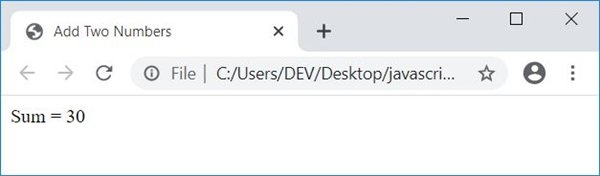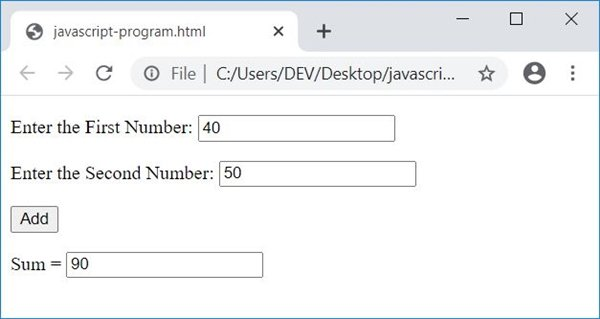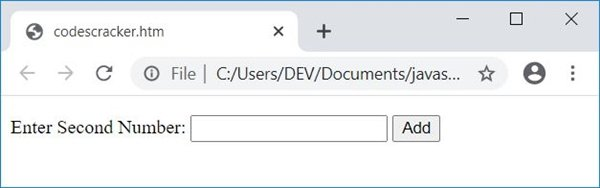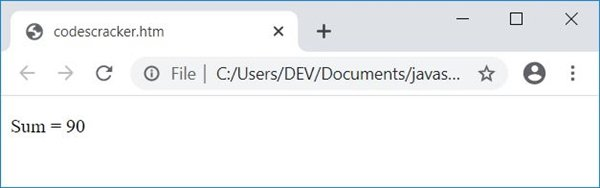# JavaScript Program to Add Two Numbers

In this article, you will learn and get code for the addition of two numbers in JavaScript. The program is created in the following ways:

• Simple JavaScript Code to Add Two Numbers
• Add two numbers using a form and a text box.
• Receive input from the user one by one.

## Add two numbers in JavaScript

Here is the simplest JavaScript code to add two numbers.

```var numOne = 10;
var numTwo = 20;
var sum = numOne+numTwo;```

As you can see from the above three lines of JavaScript code, the value 10 gets initialized to numOne. So numOne=10. And the value 20 gets initialized to numTwo. So numTwo=20. Now the statement numOne+numTwo, 10+20, or 30 gets initialized to sum. So sum=30, which is the addition of two numbers stored in numOne and numTwo.

On the same concept as mentioned above, I'm going to create another JavaScript code that also adds two numbers and displays the result as an HTML output.

## JavaScript: Add Two Numbers in HTML

Here is the JavaScript program that adds two numbers and displays the addition result on a webpage using HTML:

```<!doctype html>
<html>
<script>
var numOne = 10;
var numTwo = 20;
var sum = numOne+numTwo;
document.write("Sum = " + sum);
</script>
<body>

</body>
</html>```

Save this code in a file with .html extension. Open the file in a web browser. Here is the output you will see:Note: The document.write() method writes the value passed in its braces () into an HTML output.

## JavaScript: Add Two Numbers Using a Form and TextBox

This is the actual program to add two numbers in JavaScript using forms and text boxes. As this program allows the user to input the data. On the basis of user input, JavaScript code output the addition result.

```<!doctype html>
<html>
<script>
{
var numOne, numTwo, sum;
numOne = parseInt(document.getElementById("first").value);
numTwo = parseInt(document.getElementById("second").value);
sum = numOne + numTwo;
}
</script>
<body>

<p>Enter the First Number: <input id="first"></p>
<p>Enter the Second Number: <input id="second"></p>

</body>
</html>```

Here is its sample run with user input: 40 as the first number and 50 as the second number:When the user clicks on the button Add, then a function named add() gets called. And all the statements of this function get executed. The following statement:

`numOne = parseInt(document.getElementById("first").value);`

states that an int (integer) value of an HTML element whose id is first gets initialized to numOne. And the statement:

`document.getElementById("answer").value = sum;`

states that the value of sum gets printed to an HTML element with the id answer.

#### Live Output of the Previous Program

Following is the live output of the above JavaScript code on the addition of two numbers:

Enter the First Number:

Enter the Second Number:

Sum =

### Get user input one by one

Let's create one more interesting JavaScript program that does the same job as the previous program, that is, receives two numbers from the user and outputs its addition result.

This program receives input from the user in such a way that the user is allowed to input one at a time.

```<!doctype html>
<html>
<script>
var numOne, numTwo, sum;
function getFirNum()
{
numOne = parseInt(document.getElementById("first").value);
if(numOne)
{
temp = document.getElementById("paraOne");
temp.style.display = "none";
temp = document.getElementById("paraTwo");
temp.style.display = "block";
}
}
function getSecNum()
{
numTwo = parseInt(document.getElementById("second").value);
if(numOne && numTwo)
{
temp = document.getElementById("paraOne");
temp.style.display = "none";
temp = document.getElementById("paraTwo");
temp.style.display = "none";
sum = numOne + numTwo;
temp = document.getElementById("paraThree");
temp.style.display = "block";
document.getElementById("res").innerHTML = sum;
}
}
</script>
<body>

<p id="paraOne">Enter First Number: <input id="first">
<button onclick="getFirNum()">Enter</button></p>
<p id="paraTwo" style="display:none;">Enter Second Number: <input id="second">
<p id="paraThree" style="display:none;">Sum = <span id="res"></span></p>

</body>
</html>```

Here is the snapshot that shows the initial output produced by the above JavaScript program on the addition of two numbers entered by the user:Now enter the first number, say 50, and click on the "Enter" button. Here is the output after performing this:Now enter the second number, say 40, and click on the "Add" button. Here is the output that shows the addition result of the two numbers entered:The following code:

`style="display:none;"`

is a CSS code that hides an HTML element where it is included. Because it is included in a p (paragraph) tag whose id is paraTwo, this paragraph gets hidden initially. The following JavaScript code:

`temp.style.display = "block";`

states that, an HTML element whose id is stored in temp variable, gets visible after executing this statement. And the following statement:

`temp.style.display = "none";`

works same as previous. The only difference is, this statement hides an HTML element. And the JavaScript code:

`document.getElementById("res").innerHTML = sum;`

states that the content of an HTML element with id res gets replaced with the value of sum.

#### Live Output of the Previous Program

This is another live output produced by the previous JavaScript program:

Enter First Number:

JavaScript Online Test

« Previous Program Next Program »